Courses

# Irodov Solutions: Electromagnetic Induction Maxwell’s Equations- 2 Notes | EduRev

## NEET : Irodov Solutions: Electromagnetic Induction Maxwell’s Equations- 2 Notes | EduRev

The document Irodov Solutions: Electromagnetic Induction Maxwell’s Equations- 2 Notes | EduRev is a part of the NEET Course Physics For JEE.
All you need of NEET at this link: NEET

Q. 310. A long solenoid of cross-sectional radius a has a thin insulated wire ring tightly put on its winding; one half of the ring has the resistance η times that of the other half. The magnetic induction produced by the solenoid varies with time as B = bt, where b is a constant. Find the magnitude of the electric field strength in the ring.

Solution. 310. The changing magnetic field will induce an e.m.f. in the ring, which is obviously equal, in the two parts by symmetry (the e.m.f. induced by electromagnetic induction does not depend on resistance). The current, that will flow due to this, will be different in the two parts. This will cause an acceleration of charge, leading to the setting up of an electric field E which has opposite sign in the two parts. Thus,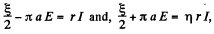where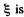the total induced e.m.f. From this,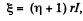and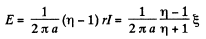But by Faraday's law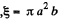so,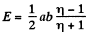Q. 311. A thin non-conducting ring of mass m carrying a charge q can freely rotate about its axis. At the initial moment the ring was at rest and no magnetic field was present. Then a practically uniform magnetic field was switched on, which was perpendicular to the plane of the ring and increased with time according to a certain law B (t). Find the angular velocity ω of the ring as a function of the induction B (t).

Solution. 311. Go to the rotating frame with an instantaneous angular velocity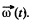In this frame, a Coriolis force,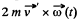acts which must be balanced by the magnetic force,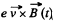Thus,

(It is assumed that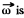small and varies slowly, so ω2 and ω can be neglected.)

Q. 312. A thin wire ring of radius a and resistance r is located inside a long solenoid so that their axes coincide. The length of the solenoid is equal to l, its cross-sectional radius, to b. At a certain moment the solenoid was connected to a source of a constant voltage V. The total resistance of the circuit is equal to R. Assuming the inductance of the ring to be negligible, find the maximum value of the radial force acting per unit length of the ring.

Solution. 312. The solenoid has an inductance,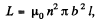where n = number of turns of the solenoid per unit length. When the solenoid is connected to the source an e.m.f. is set up, which, because of the inductance and resistance, rises slowly, according to the equation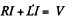This has the well known solution,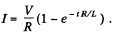Corresponding to this current, an e.m.f. is induced in the ring. Its magnetic field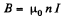in the solenoid, produces a force per unit length,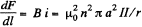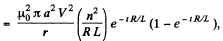acting on each segment of the ring. This force is zero initially and zero for large t. Its maximum value is for some finite t. The maximum value of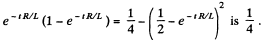So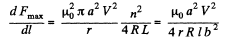Q. 313. A magnetic flux through a stationary loop with a resistance R varies during the time interval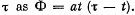Find the amount of heat generated in the loop during that time. The inductance of the loop is to be neglected.

Solution. 313. The amount of heat generated in the loop during a small time interval dt,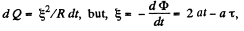So,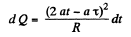and hence, the amount of heat, generated in the loop during the time interval 0 to τ.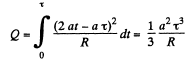Q. 314. In the middle of a long solenoid there is a coaxial ring of square cross-section, made of conducting material with resistivity p. The thickness of the ring is equal to h, its inside and outside radii are equal to a and b respectively. Find the current induced in the ring if the magnetic induction produced by the solenoid varies with time as B = βt, where β is a constant. The inductance of the ring is to be neglected.

Solution. 314. Take an elementary ring of radius r and width dr. The e.m.f. induced in this elementary ring is πr2β.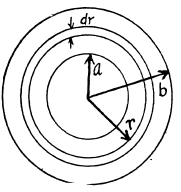Now the conductance of this ring is.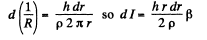Integrating we get the total current,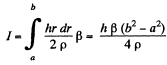Q. 315. How many metres of a thin wire are required to manufacture a solenoid of length l0  = 100 cm and inductance L = 1.0 mH if the solenoid's cross-sectional diameter is considerably less than its length?

Solution. 315. Given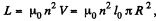where R is the radius o f the solenoid.

Thus,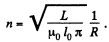So, length of the wire required is,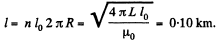Q. 316. Find the inductance of a solenoid of length l whose winding is made of copper wire of mass m. The winding resistance is equal to R. The solenoid diameter is considerably less than its length.

Solution. 316. From the previous problem, we know that,

l' = length of the wire needed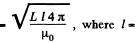length of solenoid here.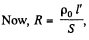(where S = area of crossection of the wire. Also m = p S l')

Thus,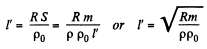where p0 = resistivity of copper and p = its density.

Equating,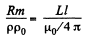or,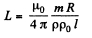Q. 317. A coil of inductance L = 300 mH and resistance R = 140 mΩ is connected to a constant voltage source. How soon will the coil current reach η = 50% of the steady-state value?

Solution. 317. The current at time t is given by,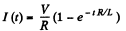The steady state value is,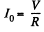and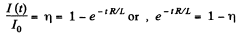or,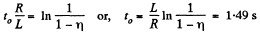Q. 318. Calculate the time constant τ of a straight solenoid of length l = 1.0 m having a single-layer winding of copper wire whose total mass is equal to m = 1.0 kg. The cross-sectional diameter of the solenoid is assumed to be considerably less than its length.
Note. The time constant ti is the ratio LIR, where L is inductance and R is active resistance.

Solution. 318. The time constant τ is given by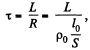where, p0 = resistivity, l0 = length of the winding wire, S = cross section of the wire. But

But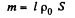So eliminating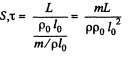From problem Q.315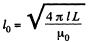(note the interchange of l and l0 because of difference in notation here.)

Thus,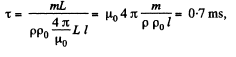Q. 319. Find the inductance of a unit length of a cable consisting of two thin-walled coaxial metallic cylinders if the radius of the outside cylinder η = 3.6 times that of the inside one. The permeability of a medium between the cylinders is assumed to be equal to unity.

Solution. 319. Between the cables, where a< r<b , the magnetic field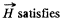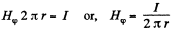So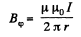The associated flux per unit length is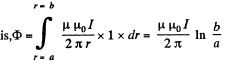Hence, the inductance per unit length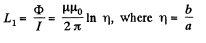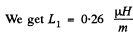Q. 320. Calculate the inductance of a doughnut solenoid whose inside radius is equal to b and cross-section has the form of a square with side a. The solenoid winding consists of N turns. The space inside the solenoid is filled up with uniform paramagnetic having permeability μ.

Solution. 320. Within the solenoid,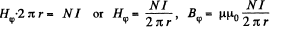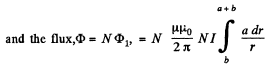Finally,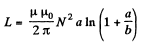Q. 321. Calculate the inductance of a unit length of a double tape line (Fig. 3.92) if the tapes are separated by a distance h which is considerably less than their width b, namely, b/h = 50.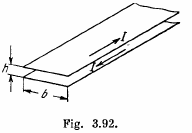Solution. 321. Neglecting end effects the magnetic field B, between the plates, which is mainly parallel to the plates, is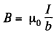(For a derivation see Q.229 b)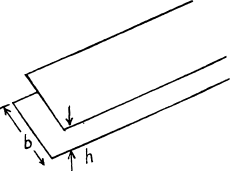Thus, the associated flux per unit length of the plates is,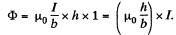So, L1 = inductance per unit length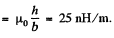Q. 322. Find the inductance of a unit length of a double line if the radius of each wire is II times less than the distance between the axes of the wires. The field inside the wires is to be neglected, the permeability is assumed to be equal to unity throughout, and η  ≫ 1

Solution. 322. For a single current carrying wire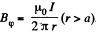For the double line cable, with current, flowing in opposite directions, in the two conductors,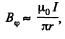between the cables, by superposition. The associated flux is,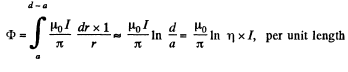Hence,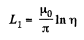is the inductance per unit length

Q. 323. A superconducting round ring of radius a and inductance L was located in a uniform magnetic field of induction B. The ring plane was parallel to the vector B, and the current in the ring was equal to zero. Then the ring was turned through 90° so that its plane became perpendicular to the field. Find:
(a) the current induced in the ring after the turn;
(b) the work performed during the turn.

Solution. 323. In a superconductor there is no resistance, Hence,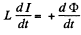So integrating,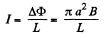because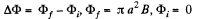Also , the work done is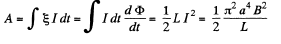Q. 324. A current I0  = 1.9 A flows in a long closed solenoid. The wire it is wound of is in a superconducting state. Find the current flowing in the solenoid when the length of the solenoid is increased by η = 5%.

Solution. 324. In a solenoid, the inductance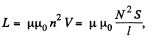where S = area of cross section of the solenoid, l = its length,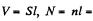total number of turns.

When the length of the solenoid is increased, for example, by pulling it, its inductance will decrease. If the current remains unchanged, the flux, linked to the solenoid, will also decrease. An induced e.m.f. will then come into play, which by Lenz’s law will try to oppose the decrease of flux, for example, by increasing the current. In the superconducting state the flux will not change and so,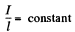Hence,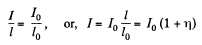Q. 325. A ring of radius a = 50 mm made of thin wire of radius b = 1.0 mm was located in a uniform magnetic field with induction B = 0.50 mT so that the ring plane was perpendicular to the vector B. Then the ring was cooled down to a superconducting state, and the magnetic field was switched off. Find the ring current after that. Note that the inductance of a thin ring along which the surface current flows is equal to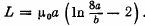Solution. 325. The flux linked to the ring can not change on transition to the superconduction state, for reasons, similar to that given above. Thus a current / must be induced in the ring, where,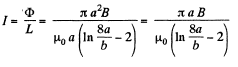Q. 326. A closed circuit consists of a source of constant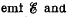a choke coil of inductance L connected in series. The active resistance of the whole circuit is equal to R. At the moment t = 0 the choke coil inductance was decreased abruptly η times. Find the current in the circuit as a function of time t.
Instruction. During a stepwise change of inductance the total magnetic flux (flux linkage) remains constant.

Solution. 326. We write the equation of the circuit as,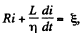for t > 0. The current at t - 0 just after inductance is changed, is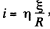so that the flux through the inductance is unchanged.

We look for a solution of the above equation in the form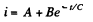Substituting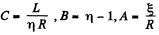Thus,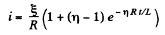Q. 327. Find the time dependence of the current flowing through the inductance L of the circuit shown in Fig. 3.93 after the switch Sw is shorted at the moment t = 0.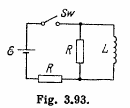Solution. 327.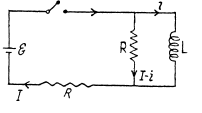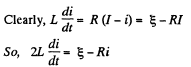This equation has the solution (as in Q.312)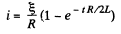Q. 328. In the circuit shown in Fig. 3.94 an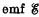, a resistance R, and coil inductances L1 and L2 are known. The internal resistance of the source and the coil resistances are negligible. Find the steadystate currents in the coils after the switch Sw was shorted.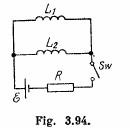Solution. 328. The equations are,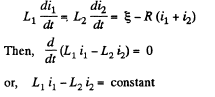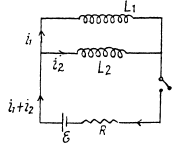But initially at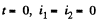so constant must be zero and at all times,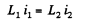In the final steady atate, current must obviously be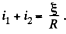Thus in steady state,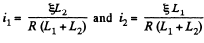Q. 329. Calculate the mutual inductance of a long straight wire and a rectangular frame with sides a and b. The frame and the wire lie in the same plane, with the side b being closest to the wire, separated by a distance l from it and oriented parallel to it.

Solution. 329. Here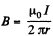at a distance r from the wire. The flux through the frame is obtained as,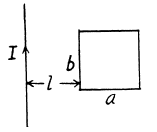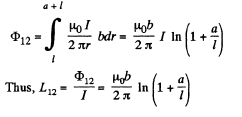Q. 330. Determine the mutual inductance of a doughnut coil and an infinite straight wire passing along its axis. The coil has a rectangular cross-section, its inside radius is equal to a and the outside one, to b. The length of the doughnut's cross-sectional side parallel to the wire is equal to h. The coil has N turns. The system is located in a uniform magnetic with permeability μ.

Solution. 330.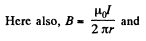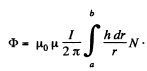Thus,Offer running on EduRev: Apply code STAYHOME200 to get INR 200 off on our premium plan EduRev Infinity!

## Physics For JEE

187 videos|516 docs|263 tests

,

,

,

,

,

,

,

,

,

,

,

,

,

,

,

,

,

,

,

,

,

;